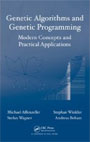Mission Statement

The book aims at both researchers and students working and studying in the field of heuristic optimization. The genetic algorithm is a metaheuristic optimization technique that has gained reputation for providing good solutions to complex problems of various fields in a short amount of time. They have been studied theoretically as well as in numerous applications to problems such as in operations research or simulation based optimization. Genetic programming constitutes a special branch of genetic algorithms where the solution to a problem is a mathematical formula which solves a classification or regression problem as good as possible. In this context the book also describes the use of advanced concepts such as offspring selection which the authors have intensively worked on over the years.

The book divides into two parts which explain theory and show results of practical application. The purpose in the theory part is to sum up the theoretical background of genetic algorithms and genetic programming approaches and analyze their behavior on simpler test problems. While in the practical part the algorithms are applied on larger problems where results are computed and interpreted.